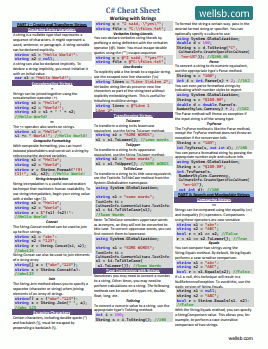# How to Use Delegates in C#

## UNDERSTANDING C# DELEGATES

by

A delegate in C# is essentially a pointer to another method. It allows your program to pass functions between methods and classes in the same way you might pass variables.

In simple terms, a delegate is like a variable that points to a method. Specifically, a delegate type holds a reference to a method that has a particular return type and particular input parameter types.

## How to use Delegates

### A Trivial Example

To begin to understand delegates, we will walk through a simple example. Start by creating a new C# Console application. Within the Program class, you will declare a delegate as follows:

``````delegate int MathDelegate(int x, int y);
``````

This delegate can be used to reference any method that both accepts two input parameters of type `int` and returns an `int` data type. For example, the delegate could potentially point to either of the following two methods:

``````public static int AddNumbers(int x, int y)
{
return x + y;
}

public static int MultiplyNumbers(int x, int y)
{
return x * y;
}
``````

Observe that both `AddNumbers()` and `MultiplyNumbers()` accept two integer parameters and return an integer, just like the declaration for the delegate `MathDelegate`. Now, in the `static void Main()` method, you could instantiate the delegate and point it to either of those two methods.

``````static void Main(string[] args)
{

Console.WriteLine("Result: {0}", myDelegate(2, 4));
}
``````

Here, we have declared a new variable, `myDelegate` of type `MathDelegate` and assigned it a method, `AddNumbers()`. We do this in the same way we would for other data types and variables in C#. Now, we can reference `myDelegate` just like we would reference the `AddNumbers()` method. We will pass it two integer parameters and expect it to return an `int`.

This example is somewhat trivial, because we could easily just reference the named method instead of assigning the method to a delegate. However, it is an important exercise to begin to understand how delegates can be used. Next, we will extend this example and begin to see the power of C# delegates.

### A Nontrivial Example

In the example above, the delegate declaration is made inside the same class as the method definitions and the execution call. A useful characteristic of delegates, however, is that delegates are not concerned about the class of the object that it references. A delegate could also be declared publicly inside another class or outside a class altogether. This would allow your program to pass around methods between classes, without unnecessarily exposing those methods outside of the class in which they are defined.

Let's extend the above example by declaring the delegate in our namespace outside of all classes.

``````delegate int MathDelegate(int x, int y);
``````

Next, create a class called `Calculations` that lists possible mathematical operations. Notice that each of these operations match the signature of the delegate declared above.

``````class Calculations
{
public static int AddNumbers(int x, int y)
{
return x + y;
}

public static int MultiplyNumbers(int x, int y)
{
return x * y;
}
}
``````

Now, create another class called `MathClass` which contains a single method named `DoMath`. This method will need to perform one of the operations defined in the `Calculations` class, but it does not know which one. In fact, no element in `MathClass` knows which operation to perform. How can we ensure that the correct `Calculation` method is called? We can simply pass our delegate to the `DoMath` method. This delegate will hold the reference to the appropriate `Calculation` method.

``````class MathClass
{
public static int DoMath(int x, int y, MathDelegate math)
{
return math(x, y);
}
}
``````

Finally, in the `Main()` method of our `Program` class, we can instantiate the `MathDelegate` object and programmatically assign a method to the new instance of the delegate type. It will then pass that delegate as a parameter to the `DoMath()` method, so the program will know which calculation to perform.

``````class Program
{
static void Main(string[] args)
{
Console.WriteLine("Result: {0}", MathClass.DoMath(2, 4, myDelegate));
}
}
``````

One Line 30, we have assign the delegate to the `addNumbers()` method of the `Calculations` class. Then, we use a composite string formatting technique to directly write the result to the console.

Notice, we have passed three parameters to the `DoMath()` method of `MathClass` - the two integers we wish to operate on and a delegate, which contains a reference to the method we wish to use. Our delegate could point to any of the same-signature operations in the `Calculations` class, and we would never have to make any changes to the `MathClass` or any of its methods. The `MathClass` is effectively isolated from the `Calculations` class. It does not know about any of the underlying logic, and it does not care.

## Where else can I use Delegates?

Delegates are powerful tools for extending existing code. As you can see in the examples above, they can be used to call a method without prior knowledge of the actual method name or even knowledge of which class the method is in. Because they allow you to transparently pass methods between classes, you can also use them to write callbacks and handle events.

Delegates are useful in programs with non-trivial user-interfaces, such as WPF applications, where you need to pass some value from a back-end process to the UI thread. A delegate can be used in this scenario to get the message or value back to the UI thread without violating standard coding principles.

Finally, it is important to note that delegates can be multicasted, using the + or += operator. This means a single delegate can reference more than one method at the same time, assuming each method has the same signature as the delegate. For example, a single delegate might be used to invoke both a Console logger method and a File logger method.

#### Don't stop learning!